# High School Math : How to find the length of the diagonal of a pentagon

## Example Questions

### Example Question #1 : How To Find The Length Of The Diagonal Of A Pentagon

Find the length of the diagonal of the following pentagon: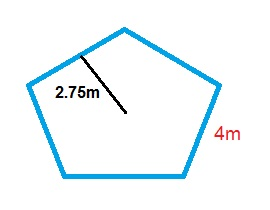Possible Answers: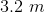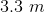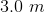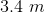Correct answer:Explanation:

Use the Pythagorean Theorem to find the length of the diagonal: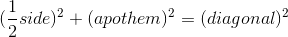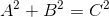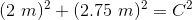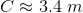### Example Question #2 : How To Find The Length Of The Diagonal Of A Pentagon

Find the length of the diagonal of the following pentagon: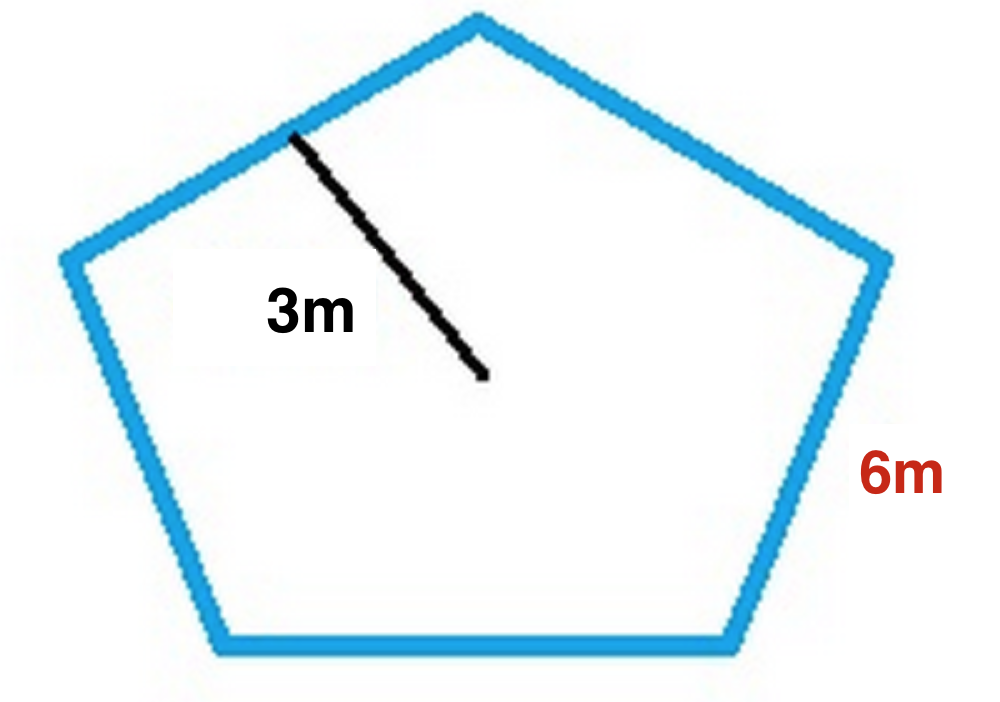Possible Answers: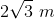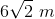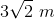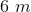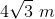Correct answer:Explanation:

Use the Pythagorean Theorem to find the length of the diagonal: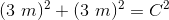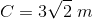### All High School Math Resources